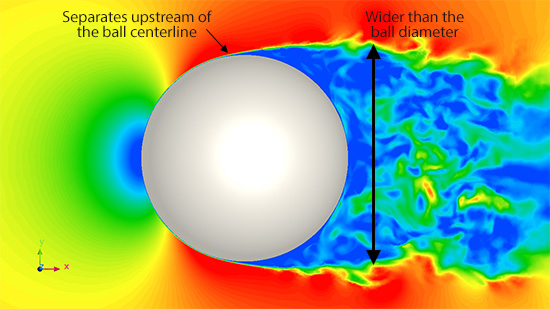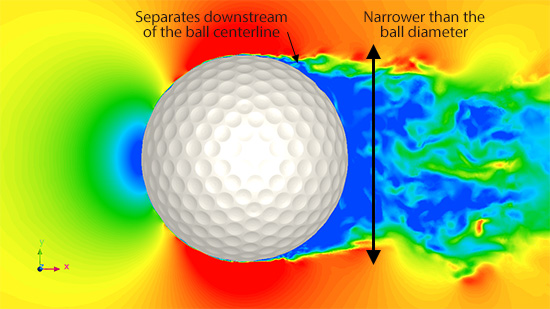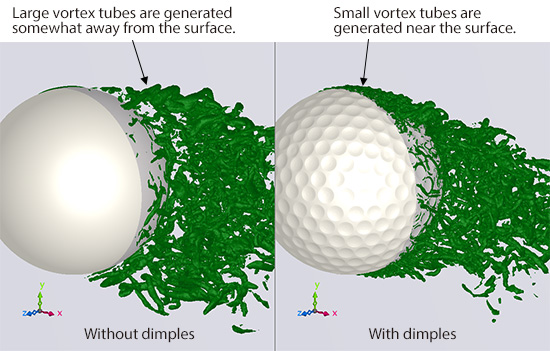# Basic Course on Turbulence and Turbulent Flow Modeling 17: 17.1 Effect of dimples

#### 17.1: Effect of dimples

In the previous column, we saw the generation of small vortices on the surface of a golf ball due to dimples. In this column, we will close in on the mechanism of how dimples lower air drag. First, let us look at the flow field around the golf ball without dimples. Figure 17.1 shows the velocity distribution on a cross section passing through the middle of the ball. The characteristic of the flow field without dimples is that the flow separates from the ball surface upstream of the ball centerline. That makes the downstream region of slow flow wider than the ball diameter. This means the air cannot stay along the ball surface because of its momentum.Figure 17.1: Flow field around the golf ball without dimples

Figure 17.2 shows the velocity distribution with dimples. In the case with dimples, flow along the ball surface keeps staying along the surface past the ball centerline and finally separates downstream. This makes the downstream region of slow flow narrower than the ball diameter.Figure 17.2: Flow field around the golf ball with dimples

How does this difference in velocity distribution occur? Figure 17.3 shows vortex tubes generated downstream of the ball. Note that only the vortex tubes in the right-hand side of the ball mid-plane are shown. On the left is the case without dimples. We can see that the generation of vortex tubes is initiated somewhat away from the ball surface and that relatively large vortex tubes are generated. On the other hand, in the case with dimples shown on the right, the generation of vortex tubes is initiated on the ball surface as we saw in Chapter Volume 16. The diameters of the vortex tubes are small compared to those in the case without dimples. We can infer that these many small vortex tubes generated on the ball surface act to mix the air near the ball and the fast-flowing air away from the ball, which in turn shifted the separation point toward downstream as seen in Figure 17.2. With these differences in the flow fields described above, the drag coefficient Cd without dimples is 0.53, while Cd with dimples is 0.28. This confirms numerically that the air drag becomes smaller with dimples.Figure 17.3: Vortex tubes downstream of the ball

Intuitively, it seems that the ball without dimples has a smoother surface and smaller air drag. When the surface is round like the golf ball, however, the smoother surface cannot prevent the flow separation. This creates a large region of stagnation behind the object, which results in a larger air drag. The large vortex tubes, such as the ones shown in Figure 17.3 for the case without dimples, also lead to the generation of large sounds. Surface asperities like dimples, therefore, not only lower air drag but also possibly reduce noises. Lastly, let us see an animation of vortex tubes around the golf ball.

Figure 17.4: Vortex tubes generated around the golf ball (top: with dimples, bottom: without dimples)